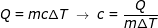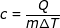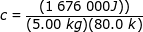• ×
السبت 6 يونيو 2020 | 05-28-2020
×

# الحرارة النوعية specific heat

When heat energy is added to a substance, the temperature will change by a certain amount. The relationship between heat energy and temperature is different for every material, and the specific heat is a value that describes how they relate.

heat energy = (mass of substance)(specific heat)(change in temperature)

Q = mc∆T

Q = heat energy (Joules, J)

= mass of a substance (kg)

c = specific heat (units J/kg∙K)

is a symbol meaning "the change in"

∆T = change in temperature (Kelvins, K)

Specific Heat Formula Questions:

1) The specific heat of gold is 129 J/kg∙K. What is the quantity of heat energy required to raise the temperature of 100 g of gold by 50.0 K?

Answer: The mass of gold is m = 100 g = 0.100 kg. The heat energy can be found using the formula:

Q = mc∆T

Q =(0.100 kg)(129 J/kg∙K)(50.0 K)

Q = 645 J

The energy required to raise the temperature of the piece of gold is 645 J.

2) A pot of water is heated by transferring 1676 kJ of heat energy to the water. If there is 5.00 kg of water in the pot, and the temperature is raised by 80.0 K, what is the specific heat of water?

Answer: The heat energy transferred to the water is 1676 kJ = 1 676 000 J. The specific heat can be found by rearranging the formula:c = 4190 J/kg∙K

The specific heat of water is 4190 J/kg∙K.بواسطة :
10-30-2018 12:00 صباحاً  0  0  535
التعليقات ( 0 )